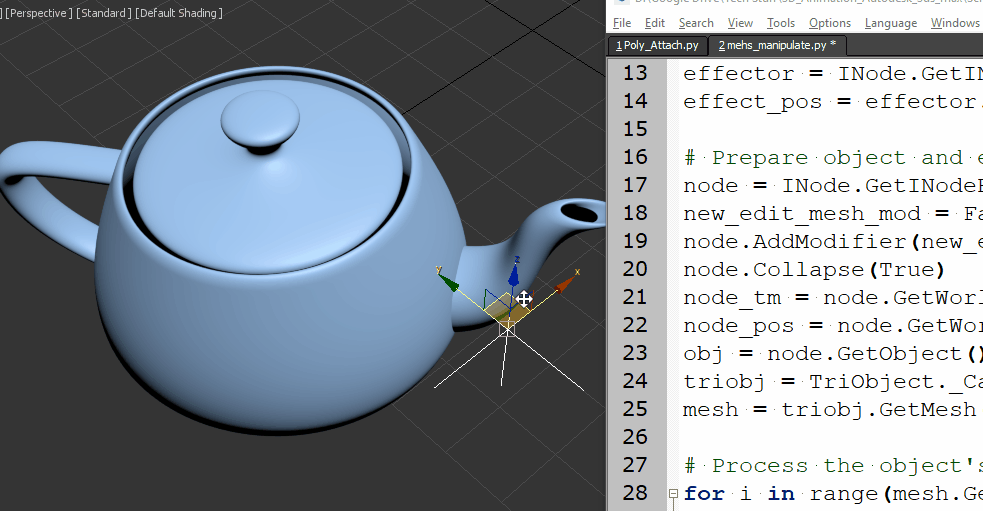## Python for 3ds max – Mesh manipulation

Software:
3ds max 2019

An example of creating a mesh ripple deformation using Python for 3ds max:Script steps:

1. Define the effect intensity and a helper point object that will serve as the effect center.
2. Collapse the object to an Editable-Mesh so its vertices will be accessible by Python.
Note that the Node‘s Object has to be cast as a TriObject, to access the object’s Mesh data.
3.  Loop through the Mesh’s vertices, get their world position, and set a new Z position as a sine function of the distance from the effect center.
```import math
from MaxPlus import Factory
from MaxPlus import ClassIds
from MaxPlus import INode
from MaxPlus import TriObject
from MaxPlus import Matrix3
from MaxPlus import Point3

# Intensity:
effecr_mult = 1.0

# Effect center:
effector = INode.GetINodeByName('Point001')
effect_pos = effector.GetWorldPosition()

# Prepare object and eccess it's mesh data:
node = INode.GetINodeByName('Teapot001')
new_edit_mesh_mod = Factory.CreateObjectModifier(ClassIds.Edit_Mesh)
node.Collapse(True)
node_tm = node.GetWorldTM()
node_pos = node.GetWorldPosition()
obj = node.GetObject()
triobj = TriObject._CastFrom(obj)
mesh = triobj.GetMesh()

# Process the object's vertices:
for i in range(mesh.GetNumVertices()):
# Get vertex in world space
vert_pos = mesh.GetVertex(i)
vert_world_pos = node_tm.VectorTransform(vert_pos)
vert_world_pos = vert_world_pos + node_pos
# Get vertex distance from effect center:
diff_vec = vert_world_pos - effect_pos
diff_vec.Z = 0
dist = diff_vec.GetLength()
# Set new vertex position:
mesh.SetVert(i,vert_pos.X,vert_pos.Y,vert_pos.Z + math.sin(dist)*effecr_mult)```

* note that when copying and pasting a script from this example, the indentation may not be pasted correctly.

Related Post:
Python for 3ds max – Animated Mesh